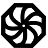# Programming-Idioms# Idiom #158 Random sublist

Create a new list y from randomly picking exactly k elements from list x.

It is assumed that x has at least k elements.
Each element must have same probability to be picked.
Each element from x must be picked at most once.
Explain if the original ordering is preserved or not.

```  allocate (sample(k))
do i=1,k
sample(i) = x(i)
end do
do i=k+1,n
call random_number(a)
j = 1 + int(i*a)
if (j .le. k) sample(j) = x(i)
end do```
`using System.Collections.Generic;`
```Random rnd = new Random();
List<int> y = x.OrderBy(r => rnd.Next()).Take(k).ToList();
```
`import std.random;`
`auto y = randomSample(x, k);`
`import "math/rand"`
```y := make([]T, k)
perm := rand.Perm(len(x))
for i, v := range perm[:k] {
y[i] = x[v]
}```
`import System.Random (randomRIO)`
```randomSample :: Int -> [a] -> IO [a]
randomSample 0 x = pure []
randomSample k x = do
i <- randomRIO (0, length x - 1)
let (a, e:b) = splitAt i x
l <- randomSample (k-1) (a ++ b)
pure (e : l)```
```const idx = x.map((item, i) => i);
while (y.length < k) {
const i = parseInt(Math.random() * idx.length, 10);
y.push(x[[idx[i]]]);
idx.splice(i, 1);
}```
`\$y = array_intersect_key(\$x, array_flip(array_rand(\$x, \$k)));`
`Types, Math`
```function RandArr(Max: Integer): TIntegerDynArray;
var
i, j, temp: Integer;
begin
SetLength(Result, Max+1);
for i := Low(Result) to High(Result) do Result[i] := i;
i := Length(Result);
while i > 0 do
begin
Dec(i);
j := RandomRange(0,i);
temp := Result[i];
Result[i] := Result[j];
Result[j] := temp;
end;
end;

var
Idx: TIntegerDynArray;
begin
Idx := RandArr(High(X));
SetLength(Y, k);
for i := 0 to k-1 do Y[i] := X[Idx];
end.```
`use List::Util qw(shuffle head);`
`my @y = head \$k, shuffle @x;`
`import random`
`y = random.sample(x, k)`
`y = x.sample(k)`
`use rand::prelude::*;`
```let mut rng = &mut rand::thread_rng();
let y = x.choose_multiple(&mut rng, k).cloned().collect::<Vec<_>>();```

#### Idiom created by

programming-idioms.org